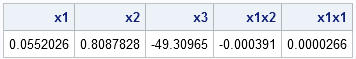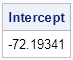Someone recently asked a question on the SAS Support Communities about estimating parameters in ridge regression. I answered the question by pointing to a matrix formula in the SAS documentation. One of the advantages of the SAS/IML language is that you can implement matrix formulas in a natural way. The SAS/IML expressions can often be written almost verbatim from the formula. This article uses a ridge regression formula from the PROC REG documentation to illustrate this feature.

### When to use ridge regression?

Ridge regression is a variant to least squares regression that is sometimes used when several explanatory variables are highly correlated. The "usual" ordinary least squares (OLS) regression produces unbiased estimates for the regression coefficients (in fact, the Best Linear Unbiased Estimates). However, when the explanatory variables are correlated, the OLS parameter estimates have large variance. It might be desirable to use a different regression technique, such as ridge regression, in order to obtain parameter estimates that have smaller variance. The trade-off is that the estimates for the ridge regression method are biased.

If X is the centered and scaled data matrix, then the crossproduct matrix X`X is nearly singular when columns of X are highly correlated. Mathematically, ridge regression adds a multiple (called the ridge parameter, k) of the identity matrix to the X`X matrix. The nonsingular matrix (X`X + kI) is then used to compute the parameter estimates.

Each value of k results in a different set of parameter estimates. There have been many papers written about how to choose the best value of k. In this article, I merely want to show how to compute the parameters for ridge regression when the ridge parameter is given.

### How to compute ridge regression in SAS

In SAS software, you can compute ridge regression by using the REG procedure. The following example from the PROC REG documentation is used to illustrate ridge regression. The RIDGE= option specifies the value(s) of the ridge parameter, k. The OUTEST= option is used to create an output data set that contains the parameter estimates for each value of k.

```/* Ridge regression example from PROC REG documentation */ data acetyl; input x1-x4 @@; x1x2 = x1 * x2; x1x1 = x1 * x1; datalines; 1300 7.5 0.012 49 1300 9 0.012 50.2 1300 11 0.0115 50.5 1300 13.5 0.013 48.5 1300 17 0.0135 47.5 1300 23 0.012 44.5 1200 5.3 0.04 28 1200 7.5 0.038 31.5 1200 11 0.032 34.5 1200 13.5 0.026 35 1200 17 0.034 38 1200 23 0.041 38.5 1100 5.3 0.084 15 1100 7.5 0.098 17 1100 11 0.092 20.5 1100 17 0.086 29.5 ;   proc reg data=acetyl outest=b ridge=0.02 noprint; model x4=x1 x2 x3 x1x2 x1x1; run;   proc print data=b(where=(_TYPE_="RIDGE")) noobs; var _RIDGE_ Intercept x1 x2 x3 x1x2 x1x1; run;```The parameter estimates for the ridge regression are shown for the ridge parameter k = 0.02.

### Implementing a matrix formula for ridge regression by using SAS/IML software

The question that was asked on the SAS Discussion Forum was about where to find the matrix formula for estimating the ridge regression coefficients. The documentation for the REG procedure includes a section that provides the formula that PROC REG uses to compute the ridge regression coefficients. The accompanying text says:

Let X be the matrix of the independent variables after centering [and scaling]the data, and let Y be a vector corresponding to the [centered]dependent variable. Let D be a diagonal matrix with diagonal elements as in X`X. The ridge regression estimate corresponding to the ridge constant k can be computed as D-1/2(Z`Z + kI)-1Z`Y.

The terms in brackets do not appear in the original documentation, but I included them for clarity. Since this is a matrix formula, let's use the SAS/IML language to implement the formula. The following SAS/IML program uses the formula to compute the ridged parameter estimates:

```/* Ridge regression coefficients computed in SAS/IML */ proc iml; use acetyl; read ALL var {x1 x2 x3 x1x2 x1x1} into X; read all var {x4} into y; close acetyl;   /* ridge regression */ xBar = mean(X); /* save row vector of means */ yBar = mean(y); /* save mean of Y */ X = X - xBar; /* center X and y; do not add intercept */ y = y - yBar; D = vecdiag(X`*X); Z = X / sqrt(D`); /* Z`Z = corr(X) */ k = 0.02; /* choose a ridge parameter */ b = (1/sqrt(D)) # inv(Z`*Z + k*I(ncol(X))) * (Z`*y); /* formula in REG doc */ print (b`)[colname={"x1" "x2" "x3" "x1x2" "x1x1"}];```So that the formula and the SAS/IML statements match each other, I have written the computation in a "natural" way, rather than in a more efficient way. See the article "Do you really need to compute that matrix inverse?" In any case, you can see that the parameter estimates that are compute from the formula agree with the estimates that are computed by PROC REG.

However, notice that the PROC IML computations do not include an intercept term. This is because the independent and dependent variables were all centered, so the intercept in these coordinates is exactly zero. To obtain the intercept in the original (uncentered) coordinates, you can use a simple formula that recovers the intercept estimate:

``` /* The ridge regression uses centered x and y. Recover the intercept: y-yBar = b1(x1-x1Bar) + ... + bn(xn-xnBar) so y = yBar-Sum(bi*xibar) + b1 x1 + ... + bn xn Consequently, b0 = yBar-Sum(bi*xibar). */ b0 = ybar - xbar * b; print b0[label="Intercept"];```### Got a matrix formula? Use SAS/IML software

As this article shows, the SAS/IML language enables you to implement matrix formulas in a natural way. Although this article implements a formula that is already built into a SAS procedure, the same ideas apply for formulas and algorithms that are not available in any SAS procedure.

So the next time that you need to implement a matrix formula that appears in a textbook, in documentation, or in a journal article, reach for the SAS/IML language!

ShareDistinguished Researcher in Computational Statistics

Rick Wicklin, PhD, is a distinguished researcher in computational statistics at SAS and is a principal developer of SAS/IML software. His areas of expertise include computational statistics, simulation, statistical graphics, and modern methods in statistical data analysis. Rick is author of the books Statistical Programming with SAS/IML Software and Simulating Data with SAS.

1.Anthony Bongard on

How would I write a subrountine that takes 2 parameters (a constant and a square matrix). Then adds that constant to the every element of the diagonal of the matrix, and passes the results back in a new parameter?

Then out puts the new matrix after the subtracted constant

I have the general idea but getting stuck on the diagonal part.

•You can ask SAS/IML programming questions at the SAS/IML Support Community.

2.proc reg data=acetyl outest=b ridge=0.02 noprint;
model x4=x1 x2 x3 x1x2 x1x1;
run;
In the above syntax can ridge value be >1?

In a model if we do ridge=5 by .5 is that right ?Or ridge can take value between 0 and 1

•The syntax is
RIDGE=STARTVALUE to STOPVALUE by INCREMENT
such as
RIDGE=1 to 5 by 0.5
The ridge parameter can be greater than 1, but it cannot be negative.

3.How do you use ridge in logistic regression models?

•I assume you mean that you want to penalize and shrink large coefficients in the logistic regression model. SAS/STAT 14.1 provides the LASSO option in PROC HPGENSELECT, which provides shrinkage of the coeffiients. If you don't have SAS/STAT 14.1, see Shtatland, Kleinman, and Cain (2004) for an alternative approach. If you have implementation/syntax questions, please post them to the SAS Support Community for Statistial Procedures. If you search the Communities, you will find a previous discussion on this issue.

•Hi Rick, I've searched SAS communities and the link you provided but they are not too hepful. Would you have a sample SAS code where you penalize and shrink coefficients in logistic regression model using L2 regularization (ridge regression) instead of L1 (Lasso)?

•